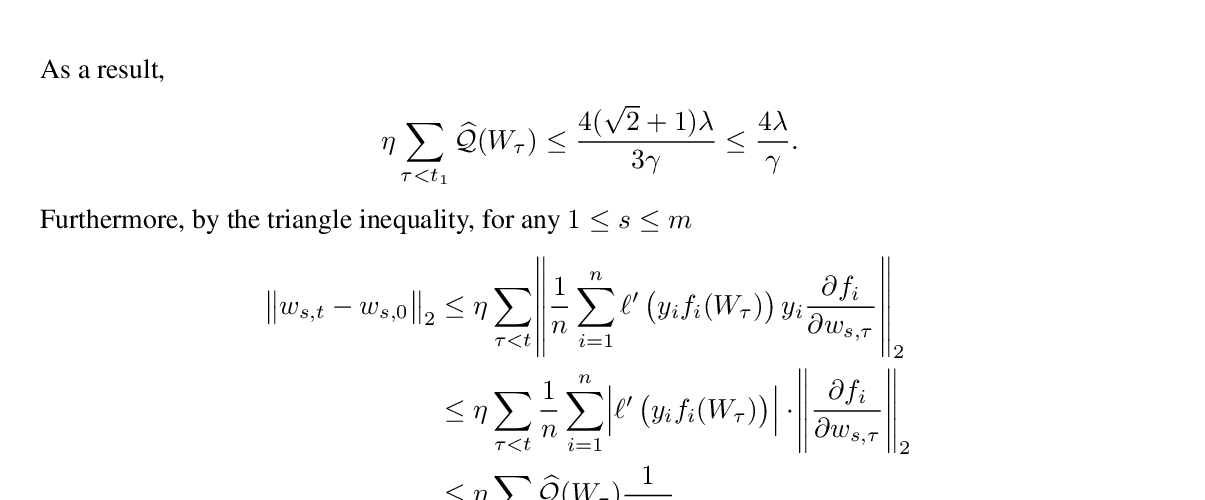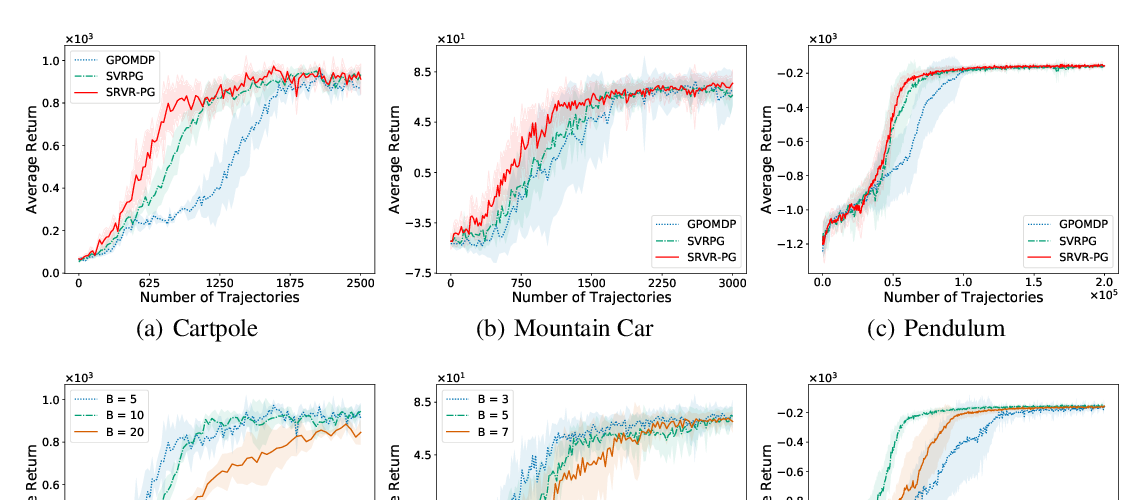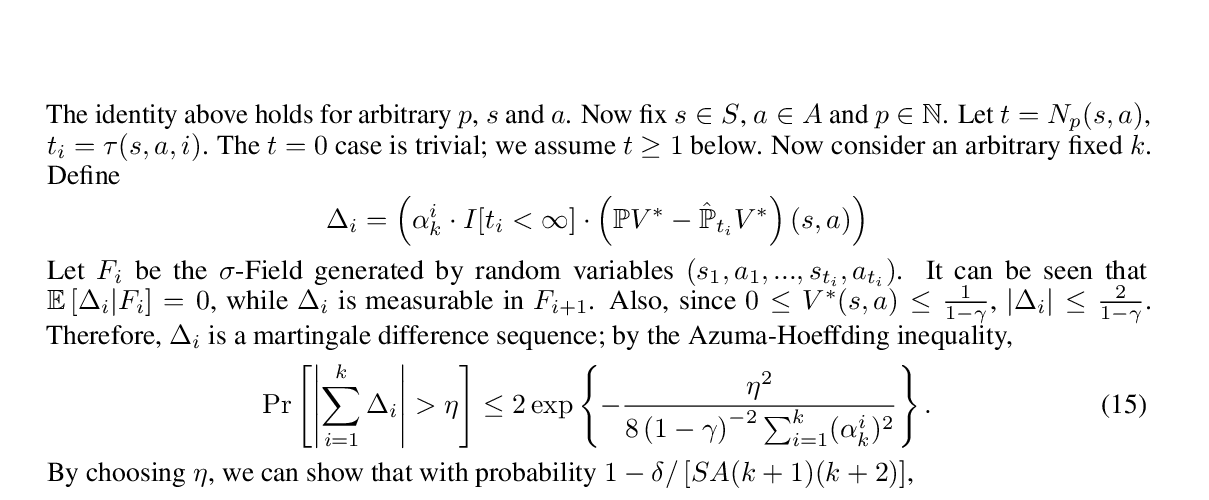## Span Recovery for Deep Neural Networks with Applications to Input Obfuscation

### Rajesh Jayaram, David P. Woodruff, Qiuyi Zhang

Abstract: The tremendous success of deep neural networks has motivated the need to better understand the fundamental properties of these networks, but many of the theoretical results proposed have only been for shallow networks. In this paper, we study an important primitive for understanding the meaningful input space of a deep network: span recovery. For $k<n$, let $\mathbf{A} \in \mathbb{R}^{k \times n}$ be the innermost weight matrix of an arbitrary feed forward neural network $M: \mathbb{R}^n \to \mathbb{R}$, so $M(x)$ can be written as $M(x) = \sigma(\mathbf{A} x)$, for some network $\sigma: \mathbb{R}^k \to \mathbb{R}$. The goal is then to recover the row span of $\mathbf{A}$ given only oracle access to the value of $M(x)$. We show that if $M$ is a multi-layered network with ReLU activation functions, then partial recovery is possible: namely, we can provably recover $k/2$ linearly independent vectors in the row span of $\mathbf{A}$ using poly$(n)$ non-adaptive queries to $M(x)$. Furthermore, if $M$ has differentiable activation functions, we demonstrate that \textit{full} span recovery is possible even when the output is first passed through a sign or $0/1$ thresholding function; in this case our algorithm is adaptive. Empirically, we confirm that full span recovery is not always possible, but only for unrealistically thin layers. For reasonably wide networks, we obtain full span recovery on both random networks and networks trained on MNIST data. Furthermore, we demonstrate the utility of span recovery as an attack by inducing neural networks to misclassify data obfuscated by controlled random noise as sensical inputs.

## Similar Papers

##### Polylogarithmic width suffices for gradient descent to achieve arbitrarily small test error with shallow ReLU networks
###### Ziwei Ji, Matus Telgarsky,##### Sample Efficient Policy Gradient Methods with Recursive Variance Reduction
###### Pan Xu, Felicia Gao, Quanquan Gu,##### Q-learning with UCB Exploration is Sample Efficient for Infinite-Horizon MDP
###### Yuanhao Wang, Kefan Dong, Xiaoyu Chen, Liwei Wang,